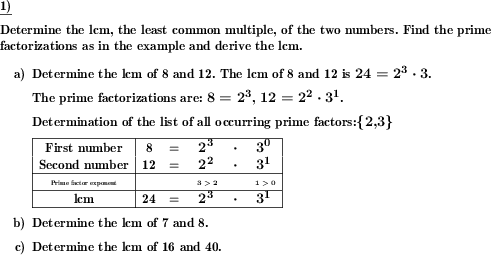Custom math worksheets at your fingertips# Details for problem "lcm derived via prime factorization"

Quickname: 3333

Elementary School, Primary School, Junior High School, Middle School, High School.

## Summary

The lcm is to be derived for two given numbers.

## Example## Description

Problems are presented for the detailed calculation of the least common multiple (lcm). The number space from which the lcm comes is adjustable. The number of problems can also be selected.

In the task, first the determination of the prime factorization for each number is required, then the derivation of the lcm. In the solution this is shown step by step.

If desired, the first problem can be output with an example solution.

Download free printable worksheets for this math problem here. The worksheet contains the problems only, the solution sheet includes the answers. Just click on the respective link.

•Worksheet 1Solution sheet with answers
•Worksheet 2Solution sheet with answers
•Worksheet 3Solution sheet with answers

If you can not see the solution sheets for download, they may be filtered out by an ad blocker that you may have installed. If this is the case, please allow ads for this page and reload the page. The solution sheets will then reappear.

• Do these sample worksheets do not really fit?
• Do you need more math worksheets, with a different level of difficulty?
• Would you like to combine different problems on a worksheet and adjust them to your needs?
• As a teacher, you can put together your own worksheets using the automatically generated math problems provided.
With a free initial credit, you can start creating your own math worksheets in a few minutes.

You can try it for free! Register here, to create custom worksheets now!

## Customization options for this problem

Parameter
Possible values
Number of problems
1, 2, 3, 4, 5, 6, 7, 8, 9, 10
Number range of product
50, 80, 100, 200, 500, 1000
Sample problem
Yes, No

## Similar problems

Remark
Description
Also as a simple mixed gcd & lcm problem using lists of divisors and multiples
For two given numbers, the gcd and lcm are determined by comparing lists of multiples or divisors